# Bohr’s Atomic Model

Niels Bohr proposed a hypothesis about electrons in a hydrogen atom. This is a model of the atomic structure where electrons travel around the nucleus in well-defined orbits determined by quantum conditions. A transition from a higher to lower orbit releases quantized energies of light, which would explain the light spectrum emitted by an element. This is known as Bohr's Atomic Theory.

Important postulates of Bohr’s atomic theory are:

1. Electrons revolve around the nucleus in circular path and are known as Orbits or Energy Level.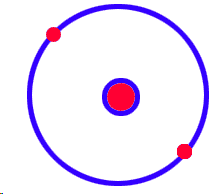2. Energy of an electron in one of its allowed orbits is fixed and can not absorb or radiate energy.

3. While Jumping electron jumps from a lower to a higher energy level which absorbs a definite amount of energy.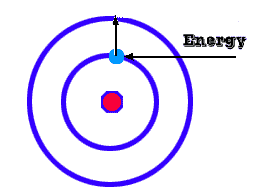4. While Jumping electron jumps from a higher to a lower energy level which also absorbs a definite amount of energy.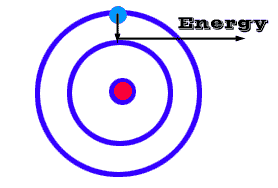5. Energy released or absorbed by an electron is equal to the energy difference between the two orbits.

Let an electron jumps from a higher energy level E2 to a lower energy level E1.The energy is emitted in the form of light .Amount of energy released is given by: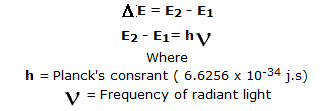6. Spectrum of light emitted from an electron is a Line Spectrum.

7. Angular momentum of an electron is given by: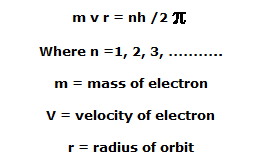Post By : Divya SIngh 04 Jan, 2020 3456 views Chemistry